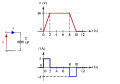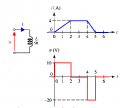# Inductors and Capacitors: different types of opposition

#### antennaboy

Joined Jan 31, 2008
48
Hello Forum,

I need a clarification about the different behavior of an inductor and a capacitor. A capacitor is a passive component that exhibits significant capacitance C. An inductor is a passive component that exhibits significant inductance L.

Inductance is defined as the property of a circuit to oppose changes in current. Capacitance is the property of a circuit to oppose changes in voltage.

Inductor: when a time-change in the main current takes place, a counter voltage develops across the inductor. This counter voltage causes an opposing current that reduces the main current (only during the main current time change). Capacitor: the main current (supplied by the voltage source) is initially large and as the capacitor gets progressively charged the main current is exponentially reduced because of the counter voltage on the capacitor. When the main current is reduced the counter voltage on the capacitor wants to keep it going and not let it die down...

So an inductor and a capacitor do have a different behavior but both generate a counter voltage which produces an opposing current limiting the main current in the circuit. So why do we say that inductance opposes changes in current and capacitance opposes changes in voltage when both produce counter voltages that in different ways oppose the main current from the voltage supply? For the capacitor, changes in voltage across which component? Not the capacitor voltage...I guess the intended load

Thanks,
Antennaboy

#### Jony130

Joined Feb 17, 2009
5,440
The capacitor oppose the change in voltage by supplying or drawing current. Capacitor current is proportional the rate of voltage change across it (proportional to how quickly the voltage across capacitor is changing). The faster the voltage change (frequency of a AC signal is high) the large the current flow through capacitor.
I = C * dV/dt
This means that to sustain current through a capacitor the applied voltage must change. The more rapidly voltage changes the larger the current. On the other hand if voltage is kept constant no current will flow no matter how large the voltage.
Likewise if the current through a capacitor is found to be zero, this means that the voltage across it must be constant, not necessarily zero.The inductors oppose the change in current by its induced voltage.
As you should know the voltage across the coil is proportional to the rate of change in current
V = L * dI/dt
This equation indicates that inductance voltage depends not on current which actually flows through the inductance, but on its rate of change. This means that to produce the voltage across an inductance, the applied current must change. If the current is kept constant, no voltage will be induced, no matter how large the current. Conversely, if it is found that the voltage across an inductance is zero this means that the current must be constant but not necessary zero.#### alfacliff

Joined Dec 13, 2013
2,458
Capacitors store voltage, inductors storre current. an inductor stores cureent in a magnetic field, when the current through the inductor changes, the magnetic field changes. if you remove or reduce the current, the magnetic field reduces, inducing current in the inductor, thus supplying current to the load. a capacitor stores power in an electric field, when ou change the voltage across the capscitor, the capacitor supplies some of the stored energy freom the stored field.

#### antennaboy

Joined Jan 31, 2008
48
Hi Jony130, thanks a lot for the infos and patience.

The source voltage and capacitor voltage are not the same at the same instant of time. There is always a delay (same goes for inductor?).

The two equations are clear:
Capacitor: I = C * dV/dt (I is the current through the capacitor and also the current in rest of the circuit. V is the voltage across the capacitor, not the voltage of the source)

V = L * dI/dt (V is the voltage across the inductor and I is the current through the inductor, which is the same as the current flowing in the rest of the series circuit made of source+inductor)

As far as the statement "Inductance opposes changes in current and capacitance opposes changes in voltage" , let me see if I have it right: In the case of a time-changing AC voltage source connected to a capacitor, the capacitor behaves in such a way to oppose the temporal changes in voltage of the voltage source and maintain the status quo (the "current through the capacitor is the Maxwell's displacement current between the plates where the dielectric is). A time change in the source voltage causes a time change in the capacitor voltage that tries to limit the source voltage change.

An inductor opposes changes in the circuit current produced by the voltage source: if the current wants to rise the inductor opposes that trying to limit it, and if the current wants to decrease the inductor opposes that by trying to sustain the current...

thanks,
antennaboy

#### Jony130

Joined Feb 17, 2009
5,440
Delay? If you connect a capacitor across a voltage source, how can you have any "delay" in this situation?

#### antennaboy

Joined Jan 31, 2008
48
What I mean is that the charges on the capacitor plates don't instantaneously adjust to the changes in the voltage source.

At an arbitrary time t, the voltage source and the capacitor source are not exactly the same. They become the same after a certain interval of time....Am I really off?

#### Jony130

Joined Feb 17, 2009
5,440
You are not off. The capacitor voltage can change instantaneously during transient, but that would mean that the current would be extremely large - infinitely large. But on steady state the capacitor voltage will reach V source no matter if it is DC or AC circuit.

#### nsaspook

Joined Aug 27, 2009
10,929
At an arbitrary time t, the voltage source and the capacitor source are not exactly the same. They become the same after a certain interval of time....Am I really off?
No, you're not off but are moving beyond circuit theory where the assumptions are zero length conductors, instantaneous conduction speed and perfect sources. Real world nothing changes instantaneously but things can happen simultaneously electrically.

#### MrAl

Joined Jun 17, 2014
9,797
Hello antennaboy,

Rather than try to understand each component by voltage alone it is more worthwhile to look at each as they appear to work in the real world. This means it is beneficial to look at the capacitor as "current driven" and the inductor as "voltage driven", when we are looking at circuits where one thing (current or voltage) is allowed to change instantaneously.

This brings us to the ideas of cause and effect.
For the capacitor, looking at the voltage as the effect, the current is the cause of that effect.
For the inductor, the current is the effect, and the voltage is the cause of that effect.

This means that connecting a non zero voltage source across an inductor begins to start a current flow though it, and connecting a non zero current source to a capacitor begins to start a voltage that builds up across it. So we look at each component a little differently, as to what is causing an effect and what that effect is. The inductor and capacitor have opposite roles because one is driven with a current and one is driven with a voltage.

Can we do the opposite: like drive the capacitor with a voltage?
We can never drive the capacitor with a non zero voltage that changes from one level to another level instantaneously, even if that second level is lower than the first. We can however drive it with a voltage that changes gradually from one level to the next, such as a ramp or sinusoid, but it is harder to understand the basic operation of the capacitor if we jump into that kind of forcing function right away. We should hold off on that kind of voltage or current source.

So the capacitor changes voltage gradually when we apply a current, and the inductor changes current gradually when we apply a voltage across it.

Another property of these two is that they are able to store energy. That is a very important property for an application like a power supply.